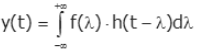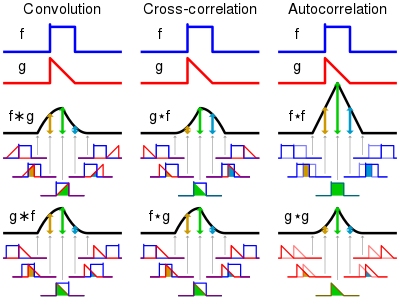# Question:How do I implement the convolution integral of 2 continuous signals in MapleSim?

## Question:How do I implement the convolution integral of 2 continuous signals in MapleSim?

Hi there!

I'm attempting to develop a custom library in MapleSim (a Modelica solver engine) that can use convolution integrals to model the hydrodynamic behaviour of floating bodies. The convolution integral is mathematically represented as follows:And the equation I'm particularly interested in solving is as follows:I'm trying to solve this convolution integral in Modelica using a model that can be imported to MapleSim. So far, I've had no luck in implementing this convolution for continuous functions symbolically. I have used a numerical approach on 2 arrays using the following approach in Modelica:

``````// Modelica function
function convIntegral
input Real simTime;  // Simulation time
input Real f[:];     // Kernel function array
input Real g[:];     // Second function array

output Real h[(2*simTime) - 1];    // Output the convolution integral in the form of an array

// Define the algorithm to numerically compute the convolution integral of 2 arrays
algorithm
// Initialize the output array with zeroes
for i in 1:((2*simTime) - 1) loop
h[i] := 0;
end for;

// Iterate over the simulation time
// Recursively increment the convolution array with the pointwise product of the 2 functions
for i in 1:simTime loop
for j in 1:simTime loop
h[i+j-1] := (f[i] * g[j]) + h[i+j-1];
end for;
end for;
end convIntegral;
// End of function to compute the convolution integral``````

This works perfectly for discrete samples and I have verified it with output from Matlab's inbuilt function

``conv(A,B)  % For 2 arrays A and B``

However, I would like to implement this on 2 continuous functions and this numerical approach does not work since MapleSim does not support conversion between discrete and continuous signals.

I understand that convolution is essentially an operation between two functions, where we time-flip one of the functions (kernel) and then slide it across the other function while measuring the bounded area and outputting that as the result of the convolution. I include this image from Wikipedia that sums up convolution: (Not including links as they mark questions as spam)I've tried implementing this in Modelica using the following code:

``````// Model to perform convolution on 2 continuous functions
model ConvolutionalIntegral

extends Modelica.Blocks.Icons.Block;

// Define model variables
Real kernelFunction = (e ^ (-0.5 * time)) * cos(0.5 * time);  // I've taken an example of a function I might use
Real kernelFunctionFlipped = (e ^ (-0.5 * (T_sim - time))) * cos(0.5 * (T_sim - time));  // I've flipped the kernel function about the vertical axis by converting the (time) variable to (T_sim - time) where (T_sim) is a variable storing the simulation duration
Real secondFunction;  // The other function for the convolution
Real convolutionIntegralOutput;  // Function to store the output

equation
// Convolution implementation
der(convolutionIntegralOutput) = kernelFunctionFlipped * secondFunction;

// Final equation to solve
der(secondFunction) + convolutionIntegralOutput = 0;
// An example of a differential equation I'd like to solve involving the convolution integral

end ConvolutionIntegral;``````

I had hoped that this would yield the output of the convolution since I'm essentially multiplying the time-flipped kernel and the other function and then integrating them over time. However, the output does not provide the expected result and it appears that Modelica interprets my code to mean that I'm integrating the pointwise product of these 2 functions over time instead of sliding the kernel over the other function.

I'd appreciate it if you could take a look at my code and my approach to solving the convolution integral symbolically, and point out where I'm making a mistake and what a possible fix might be.

Thank you!﻿